# JP5407087B1 - Fraction divider circuit - Google Patents

## Info

Publication number
JP5407087B1
JP5407087B1 JP2013158244A JP2013158244A JP5407087B1 JP 5407087 B1 JP5407087 B1 JP 5407087B1 JP 2013158244 A JP2013158244 A JP 2013158244A JP 2013158244 A JP2013158244 A JP 2013158244A JP 5407087 B1 JP5407087 B1 JP 5407087B1
Authority
JP
Japan
Prior art keywords
clock
cts
circuit
output
counter
Prior art date
Legal status (The legal status is an assumption and is not a legal conclusion. Google has not performed a legal analysis and makes no representation as to the accuracy of the status listed.)
Expired - Fee Related
Application number
JP2013158244A
Other languages
Japanese (ja)
Other versions
JP2015019343A (en
Inventor

Original Assignee

Priority date (The priority date is an assumption and is not a legal conclusion. Google has not performed a legal analysis and makes no representation as to the accuracy of the date listed.)
Filing date
Publication date
Application filed by 邦彦 公山 filed Critical 邦彦 公山
Priority to JP2013158244A priority Critical patent/JP5407087B1/en
Application granted granted Critical
Publication of JP5407087B1 publication Critical patent/JP5407087B1/en
Publication of JP2015019343A publication Critical patent/JP2015019343A/en
Expired - Fee Related legal-status Critical Current

• 244000241601 filaree Species 0.000 claims abstract description 139
• 229920000729 poly(L-lysine) polymers Polymers 0.000 description 7
• 241001417517 Scatophagidae Species 0.000 description 3
• 101710071007 CREM Proteins 0.000 description 2
• 101710072306 HBT1 Proteins 0.000 description 2
• 281000137097 Multi Media Interface companies 0.000 description 1
• 239000003990 capacitor Substances 0.000 description 1
• 238000010586 diagrams Methods 0.000 description 1
• 230000004048 modification Effects 0.000 description 1
• 238000006011 modification reactions Methods 0.000 description 1

## Images

••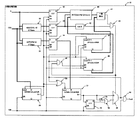•••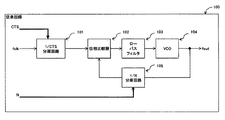•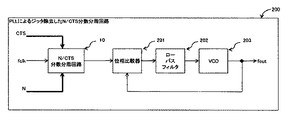•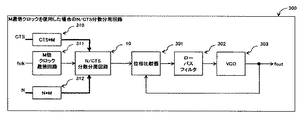•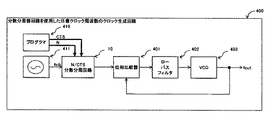•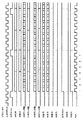••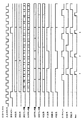•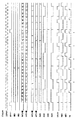••## Classifications

• HELECTRICITY
• H03BASIC ELECTRONIC CIRCUITRY
• H03KPULSE TECHNIQUE
• H03K23/00Pulse counters comprising counting chains; Frequency dividers comprising counting chains
• H03K23/64Pulse counters comprising counting chains; Frequency dividers comprising counting chains with a base or radix other than a power of two
• H03K23/68Pulse counters comprising counting chains; Frequency dividers comprising counting chains with a base or radix other than a power of two with a base which is a non-integer
• HELECTRICITY
• H03BASIC ELECTRONIC CIRCUITRY
• H03KPULSE TECHNIQUE
• H03K21/00Details of pulse counters or frequency dividers
• H03K21/08Output circuits
• H03K21/10Output circuits comprising logic circuits

## Abstract

Provided is a fractional frequency dividing circuit that can reduce jitter by arranging clock edges with little deviation.
[Solution]
When the CTS / N quotient is CTSquo and the remainder of CTS / N is CTSrem, the fractional frequency dividing circuit 10 of the present invention has a down counter 20 that divides the input clock by 1 / CTS, and the input clock is 1 / CTSquo. A down counter 51 that divides the frequency, a clock adding circuit 52, 53, and 54 that adds one clock to the output of the down counter 51, an up counter 44 that counts the number of CTSqua cycles with the carry of 51 or the output of the clock adding circuit, An up counter 46 that counts CTSrem, a coincidence detection circuit 41 that determines whether or not an integer multiple of N / CTSrem matches the counter value, and a clock addition circuit when a coincidence is detected by the coincidence detection circuit 41 Output from 52, 53, 54 is output as an output clock, and no match is detected. If, and a selector circuit 55 for outputting the output from the down counter 51 as the output clock.
[Selection] Figure 2

## Description

The present invention relates to a fractional frequency dividing circuit used in a clock generation circuit that generates a clock frequency expressed by a fractional ratio with respect to an original oscillation clock by using a digital frequency divider.
In HDMI (High-Definition Multimedia Interface), the sink device receives parameters CTS and N to reproduce the audio clock. CTS and N represent the frequency division ratio between the TMDS clock and the audio clock, and the sink device performs fractional frequency division using these parameters and reproduces the audio clock from the TMDS clock. As prior art relating to the fractional frequency dividing circuit, there are Patent Documents 1 to 9.
Japanese Patent Laid-Open No. 54-025658 Japanese Patent Laid-Open No. 02-096429 Japanese Patent Laid-Open No. 02-271717 Japanese Patent Laid-Open No. 08-242165 JP-A-11-098007 JP 2006-174197 A Japanese Patent Laid-Open No. 03-155219 Japanese Patent Laid-Open No. 04-083425 JP 2009-267651 A
In input clock fclk and output clock fout,
Suppose there is a relationship. As shown in FIG. 1, when N clocks exist in a period obtained by dividing fclk by 1 / CTS, the average frequency of fout0 satisfies the above relational expression. However, when the frequency is divided as shown in FIG. 1, the edge is biased to N clocks and the jitter becomes large.
SUMMARY OF THE INVENTION Therefore, an object of the present invention is to provide a fractional frequency dividing circuit that can reduce jitter by arranging clock edges with little deviation.
When the present invention is grasped by a circuit configuration, it has the following characteristics. The present invention is a fractional frequency dividing circuit (10) capable of outputting an output clock obtained by dividing an input clock by N / CTS, which is a ratio of an integer CTS and an integer N, and the quotient of CTS / N is CTSquo. When the remainder of CTS / N is CTSrem, a first frequency dividing circuit (20) that divides the input clock by 1 / CTS and a second frequency dividing circuit (51) that divides the input clock by 1 / CTSqua. A clock adding circuit (52, 53, 54) for adding one clock to the output of the second frequency dividing circuit, and the number of cycles of the output of the second frequency dividing circuit as the carry or clock adding circuit of the second frequency dividing circuit When a match is detected by the match detection circuit, a counter (44) that counts at the output of the signal, a match detection circuit (41) that determines whether the integer multiple of N / CTSrem matches the value of the counter, Black Outputs the output from the click additional circuit as the output clock, if a match is not detected, and a selector circuit for outputting an output from the second frequency divider as the output clock.
Further, when the present invention is functionally grasped, it has the following features. The present invention is a fractional frequency dividing circuit capable of outputting an output clock obtained by dividing an input clock by N / CTS, which is a ratio of an integer CTS and an integer N, where the quotient of CTS / N is CTSqua and CTS / When the remainder of N is CTSrem, the input clock is divided by 1 / CTS, the number of CTSqua cycles is counted in the CTS clock divided by 1 / CTS, and the count value is an integral multiple of N / CTSrem. A clock having a cycle of CTSqua + 1 is output when they match, and a clock having a cycle of CTSquo is output when they do not match.
Further, the present invention is characterized in that a clock is output at any time with respect to fluctuating CTS and N in order to calculate the period of the output clock in real time for each period of input clock period × CTS.
The clock jitter of fout is reduced by making the variation of the period of fout less than or equal to one period of fclk. The variation in the calculated period is less biased and low frequency jitter is reduced. Since the period of the output clock is calculated in real time using the quotient of CTS / N and the remainder, it is possible to operate even with changing CTS and N. Any value can be calculated as long as it is an integer N equal to or less than the integer CTS.
These and other objects, features, aspects and advantages of the present invention will become more apparent from the following detailed description when taken in conjunction with the accompanying drawings.
Example of fractional division with CTS and N Example of fractional divider circuit Example of fractional division using CTS / N quotient and remainder Relation between integer quotient and rational quotient of N / CTSrem Conventional circuit A fractional frequency divider using a PLL to remove jitter Fraction divider using M-multiplied clock Clock generator with arbitrary clock frequency using fractional frequency divider Time chart when CTS = 7, N = 7 Time chart when CTS = 12, N = 4 (CTS is divisible by N) Time chart when CTS = 11, N = 3 (when there is a remainder in CTS / N and simple) Time chart when CTS = 22, N = 6 (when there is a remainder in CTS / N) Time chart when CTS = 13 and N = 7 (when the remainder of CTS / N is the maximum) Time chart when CTS = 29, N = 8 (when there is a remainder of CTS / N and the remainder is about half of N)
Hereinafter, embodiments of the fractional frequency dividing circuit according to the present invention will be described.
FIG. 2 is a circuit diagram showing an embodiment of a fractional frequency dividing circuit according to the present invention. In FIG. 2, 10 is a fractional frequency dividing circuit, 20 is a CTS count down counter, 30 is a CTS remainder arithmetic circuit, 31 is a CTS quotient arithmetic circuit, 32 is an N-value latch circuit, 33 is a CTS remainder value latch circuit, and 34 is CTS quotient latch circuit, 40 is a fout clock number comparison operation circuit, 41 is a fout clock number coincidence detection circuit, 42 is a non-zero detection circuit, 43 is a logical product circuit, 44 is an up counter for counting a fout clock, and 45 is a CTSrem Counting up counter, 46 is a logical product circuit, 50 is a logical sum circuit, 51 is a down counter for fout period, 52 is a flip-flop circuit, 53 is an inverter circuit, 54 is a logical product circuit, 55 is a 2: 1 selector circuit, Reference numeral 60 denotes an AND circuit.
As shown in FIG. 1, when the input clock fclk is divided by N / CTS, it is necessary that N clocks exist in one cycle obtained by dividing fclk by 1 / CTS. Therefore, N / CTS frequency division can be performed using the relational expression between the quotient obtained by dividing CTS by N and the remainder.
If CTSqua is the quotient when CTS is divided by N and CTSrem is the remainder, there is the following relationship.
FIG. 3 shows the relationship between CTSquo and CTSrem. When CTS is not divisible by N, N clock edges having a CTS quo period are arranged uniformly, and the clock edge does not exist in the CTSrem clock region, so the period is biased. When CTS is divisible by N, CTSrem is 0, and the period of fout1 is the CTSquo clock, so that the jitter is 0. Therefore, when the CTS is not divisible by N, it is necessary to distribute the clock edges.
The CTSrem clock regions are distributed, and clock edges are arranged in CTSrem (CTSqua + 1) clock cycles and (N-CTSrem) CTSqua clock cycles. So clock
CTSrem) Since the relational expression CTSquo is established, N / CTS frequency division is realized. Since the number of clock edges is N,
The clock fluctuation is one cycle or less of fclk.
Next, a method for arranging clock edges will be considered with reference to FIG. FIG. 4 shows a graph in which CTSrem is assigned to the vertical axis and N is assigned to the horizontal axis. For a linear function y = (CTSrem / N) x that is (1 / CTSrem) times N to (CTSrem / CTSrem) times, a rational solution that is the value of x when y is varied from 1 to CTSrem is ( 0,0) and (N, CTSrem) and the horizontal axis of the straight line drawn vertically from the intersection of the lines drawn from 1 to CTSrem of the vertical axis. For the quotient when N is divided by CTSrem, the integer quotient when it is divisible and the divisor
Here, if one scale of the horizontal axis is a CTS quo clock interval and the count value of the N counter coincides with the integer quotient of CTS / N, one clock of fclk is added in order to distribute the CTSrem clocks. Then, the interval is CTSquo or CTSquo + 1, the number of CTSquo is (N-CTSrem), and the number of CTSqua + 1 is CTSrem.
Each time the N counter counts, fclk is gated to output one clock. The output clock (fout) has N clock edges, and has (N-CTSrem) CTSqua cycle clocks and CTSrem (CTSqua + 1) cycle clocks. This
The clock fluctuation is one cycle or less of fclk. CTSqua and (CTSqua + 1) are arranged in a distributed manner with little deviation.
The fractional frequency dividing circuit that realizes the distributed clock arrangement as described above is realized by the circuit shown in FIG.
2 in FIG. 2 is a CTS count down counter. As for the value of CTS, fclk is updated every CTS clock. The CTS count down counter generates a carry signal when Q = 1, loads the CTS value, and counts down. Therefore, a 1 / CTS frequency dividing circuit (first frequency dividing circuit) is obtained. At this time, the CTS does not need to be a fixed value, and may change at any time.
Reference numeral 32 denotes an N-value latch circuit. Note that N does not have to be a fixed value, and may vary as needed. 32 latches the value of N every time the 20 down counter generates a carry signal. A latch circuit 33 holds the remainder of CTS / N. 33 latches the remainder of the CTS / N output by the 30 CTS remainder arithmetic circuits each time the 20 down counter generates a carry signal. A latch circuit 34 holds the quotient of CTS / N. 34 latches the value of the CTS / N quotient output by the 31 CTS quotient calculation circuit each time the 20 down counter generates a carry signal.
44 is an up counter for the fout clock. 44 is loaded with 1 every time the 20 down counter generates a carry signal, and the count value is incremented every time the 2: 1 selector of 55 generates 1 to calculate the number of clocks (Nnum).
Reference numeral 45 denotes a CTSrem count upcount. 45 is loaded with 1 every time the 20 down counter generates a carry signal, and the count value is incremented each time 55 2: 1 selector circuit and 43 OR circuit generates 1, and the number of CTSrem inserted. (Crem) is calculated.
The coincidence detection circuit 41 compares the comparison value of the fout clock number with the number of clocks (Nnum). If the values match, 41 outputs 1. When CTS is divisible by N, it is not necessary to distribute CTSrem because CTSrem = 0. Therefore, when CTRSrem = 0 in the 42 non-zero detection circuit, 42 outputs 0, and the output signal of the 41 coincidence detection circuit is masked by the 43 AND circuit.
Reference numeral 51 denotes a fout cycle count down counter. Each time 20 down counters generate a carry signal or 1 is output from 55 2: 1 selector circuit, the CTS / N quotient value (CTSqua) is loaded. The 51 down counter outputs 1 when the value of Q becomes equal to 1. A flip-flop circuit 52 shifts one clock by the carry signal of 51. The output of the 53 inverter circuit is an inverted signal of the carry signal of the 51 down counter. The AND circuit 54 masks the output of the 52 flip-flops when the down counter 51 outputs 1 to the carry signal, and outputs the 52 flip-flop output signals otherwise. Reference numerals 52, 53, and 54 denote clock addition circuits that add one clock to the clock output from 51.
When the select signal of the 2: 1 selector circuit of 55 is 0, the cure signal of the 51 down counter becomes the load signal, and when the select signal is 1, the signal obtained by shifting the cure signal of the 51 down counter by 1 clock is the load signal. It becomes. Therefore, when the 55 select signal is 0, the 51 down counter functions as a 1 / CTS quo divider circuit for dividing the input clock by 1 / CTS quo. When the 55 select signal is 1, the 51 down counter functions as an input clock. It functions as a 1 / (CTS quo + 1) frequency dividing circuit for dividing 1 / (CTS qua + 1). The down counter 51 will be referred to as a second frequency dividing circuit.
The number of clocks Nnum output from 44 corresponds to the integer value on the horizontal axis described in FIG.
When the select signal 1 is output, the 51 down counter functions as a 1 / (CTSqua + 1) frequency divider circuit. When they do not match or when CTRSrem = 0, the select signal 0 is output from 43 and the 51 down counter The counter functions as a 1 / CTS quo divider circuit. As a result, (N-CTSrem) clocks with a cycle of CTSqua are output, CTSqua + 1 clocks are output with CTSrem, and the clocks with a cycle of CTSquo + 1 are output in a distributed manner.
The 60 AND circuit masks fclk with a carry signal output from 55 2: 1 selector. At this time, the clock edge of the clock signal output from fout is:
The clock fluctuation is one cycle or less of fclk. CTSqua and (CTSqua + 1) are arranged in a distributed manner with little deviation.
FIG. 5 shows an embodiment of a conventional circuit. Reference numeral 100 denotes an example of a conventional circuit. 101 is a 1 / CTS frequency divider, 102 is a phase comparator, 103 is a low-pass filter, 104 is a VCO circuit, and 105 is a 1 / N frequency divider. Since the frequency dividing circuit of 101 divides fclk by 1 / CTS and multiplies it by N using the PLL configured by the circuits of 102, 103, 104, and 105.
Satisfy the relationship. The phase comparison frequency is fclk / CTS.
FIG. 6 shows an embodiment in which the jitter of the output signal of the N / CTS fractional frequency divider is removed using a PLL. Reference numeral 10 denotes an N / CTS fractional frequency dividing circuit according to the present invention. 201 is a phase comparator, 202 is a low-pass filter, and 203 is a VCO circuit. 201, 202, and 203 constitute a PLL. The high-frequency phase jitter is removed by the 202 low-pass filter. The signal output from the 10 N / CTS fractional divider is a combination of 1 / (INT (CTS / N)) or 1 / (INT (CTS / N) +1) of the fclk clock. The fluctuation of the period is equal to or less than the period of one clock of fclk.
Since the phase comparison frequency is the frequency of fout, it is higher than that of the conventional circuit shown in FIG. 5, so that the cutoff frequency of the 202 low-pass filter can be set high. If the cut-off frequency is the same as that of the conventional circuit, more phase errors can be removed, and output clock jitter is reduced. Further, since the capacitance of the capacitor constituting the low-pass filter becomes small, it can be mounted on an LSI.
FIG. 7 shows an embodiment of an N / CTS fractional frequency dividing circuit using an M multiplied clock. Reference numeral 10 denotes an N / CTS fractional frequency dividing circuit according to the present invention. Let M be a multiple that multiplies fclk. 310 is
It is. Reference numeral 303 denotes a VCO circuit. A PLL is composed of 301, 302, and 303.
Therefore, it is the same as when the clock is not multiplied. The period of the clock input to the 10 fractional frequency dividing circuit is 1 / M. Therefore, since the variation in the period of fout is not more than one cycle of the input clock, the variation in the period is also 1 / M. Therefore, the amount of phase error removed by the low-pass filter 302 can be reduced.
HDMI reproduces an audio clock by transmitting a signal with three sets of data lines and one set of clocks, and transmitting the values of CTS and N with a packet. In the present invention, it is only necessary to transmit the values of CTS and N at the same time as the clock signal. Therefore, even with S / PDIF, it is possible to perform clock recovery by changing the frequency with CTS and N. The S / PDIF transmits clock and data information through a single signal line. If the CTS and N value information is put on the user bits and the CTS and N separated on the receiving side are given to the fractional frequency dividing circuit, a clock with a frequency of any CTS and N can be reproduced.
FIG. 8 shows an embodiment of a clock generation circuit having an arbitrary clock frequency using a fractional frequency dividing circuit. Reference numeral 10 denotes an N / CTS fractional frequency divider according to the present invention. A programmer 410 sets CTS and N values. Reference numeral 411 denotes an oscillator that supplies a reference clock to fclk. 401 is a phase comparator. Reference numeral 402 denotes a low-pass filter. Reference numeral 403 denotes a VCO circuit. 401, 402, and 403 constitute a PLL. The output fclk is
Is satisfied, and the frequency of an arbitrary CTS to be set and the value of N is output. Since the fractional frequency dividing circuit calculates the period of the output signal of fout in real time, the values of CTS and N can be changed at any time.
Examples of time charts when several values are substituted for CTS, N are shown in Figs. These time charts are merely examples for helping understanding, and any integer can be used as the value of CTS, N. As shown in the time charts of FIGS. 9 to 13, it can be seen that the present embodiment operates as desired regardless of whether or not there is a remainder.
FIG. 14 is annotated for easy understanding of the present embodiment. In the time chart shown in FIG. 14, CTS = 29 and N = 8.
in this case,
CTSqua = INT (CTS / N) = 3
CTSrem = MOD (CTS, N) = 5
And the period of fout is 3 or 4. There are five CTSqua + 1 to add Fclk1 clock.
34 output is latched with CTS / N quotient = 3 and 33 output with CTS / N remainder = 5. The counter value of 20 is counted down from CTS = 29. When the count value is 1, 1 is output for 20 carry.
44 Nnum counters and 46 Crem counters load 1 when 20 carry is 1. 44 is counted up when 55 selectors output 1, and 46 is counted up when 55 selectors are 1 and the logical product of 43 is 1. For 43 outputs, 1 is output when the integer multiple of N / CTSrem (40 outputs) matches the counter value of 44, and 0 is output if they do not match.
When 43 outputs are 1, the clock is added and CTSqua + 1, and when it is 0, the cycle is CTSquo with 51 carry outputs.
By gating the input clock with the logical product of 60, the clock is output to fout.
As a result: 1. N = 8 clock edges 2. Create CTSqua + 1 by distributing CTSrem = 5. 3. Since the cycle is CTSqua = 3 or CTSquo + 1 = 4, the variation is less than one input clock cycle. CTSqua + 1 is arranged with little bias.
Although the present invention has been described in detail above, the above description is merely illustrative of the present invention in all respects and is not intended to limit the scope thereof. It goes without saying that various improvements and modifications can be made without departing from the scope of the present invention.
The present invention relates to a fractional frequency dividing circuit and can be used industrially.
10 fraction dividing circuit 20 CTS count down counter 30 CTS remainder operation circuit 31 CTS quotient operation circuit 32 N-value latch circuit 33 CTS remainder latch circuit 34 CTS quotient latch circuit 40 fout clock number comparison operation circuit 41 fout clock number coincidence detection Circuit 42 Non-zero detection circuit 43, 54, 60 AND circuit 44 fout clock count up counter 45 CTSrem count up counter 46 AND circuit 50 OR circuit 51 fout cycle down counter 52 flip-flop circuit 53 inverter circuit 55 2 1 selector circuit 100 conventional circuit 101 1 / CTS frequency dividing circuit 102, 201, 301, 401 phase comparator 103, 202, 302, 402 low-pass filter 104, 203, 303, 403 VCO circuit 105 1 / N Frequency dividing circuit 200 Fraction frequency dividing circuit using PLL with output clock jitter removed 300 Frequency dividing circuit using M multiplied clock
311 M times clock multiplier
400 Clock Generator 410 with Arbitrary Clock Frequency Using Fraction Divider Circuit Programmer 411 for Setting CTS and N Reference Clock Oscillator

## Claims (3)

1. A fractional frequency dividing circuit capable of outputting an output clock obtained by dividing an input clock by N / CTS, which is a ratio of an integer CTS and an integer N,
When the quotient of CTS / N is CTSqua and the remainder of CTS / N is CTSrem,
A first frequency divider that divides the input clock by 1 / CTS;
A second frequency divider that divides the input clock by 1 / CTS quo;
A clock adding circuit for adding one clock to the output of the second frequency dividing circuit;
A counter that counts the number of cycles of the output of the second divider circuit by the carry of the second divider circuit or the output of the clock adding circuit;
A coincidence detection circuit that determines whether or not an integer multiple of N / CTSrem and the value of the counter coincide;
A selector circuit that outputs an output from the clock adding circuit as an output clock when a match is detected by the match detection circuit, and outputs an output from the second frequency divider circuit as an output clock when no match is detected And a fractional frequency dividing circuit.
2. A fractional frequency dividing circuit capable of outputting an output clock obtained by dividing an input clock by N / CTS, which is a ratio of an integer CTS and an integer N,
When the quotient of CTS / N is CTSqua and the remainder of CTS / N is CTSrem,
The input clock is divided by 1 / CTS, and the number of CTSqua cycles is counted in the 1 / CTS-divided CTS clock. If the count value matches an integer multiple of N / CTSrem, the cycle is CTSqua + 1. A fractional frequency dividing circuit characterized by outputting a clock having a cycle of CTSquo if the clocks do not coincide with each other.
3.   The fractional frequency dividing circuit according to claim 1 or 2, wherein a clock is output at any time with respect to the changing CTS and N.
JP2013158244A 2013-07-12 2013-07-12 Fraction divider circuit Expired - Fee Related JP5407087B1 (en)

## Priority Applications (1)

Application Number Priority Date Filing Date Title
JP2013158244A JP5407087B1 (en) 2013-07-12 2013-07-12 Fraction divider circuit

## Applications Claiming Priority (2)

Application Number Priority Date Filing Date Title
JP2013158244A JP5407087B1 (en) 2013-07-12 2013-07-12 Fraction divider circuit
US14/217,654 US9143135B2 (en) 2013-07-12 2014-03-18 Fractional frequency divider circuit

## Publications (2)

Publication Number Publication Date
JP5407087B1 true JP5407087B1 (en) 2014-02-05
JP2015019343A JP2015019343A (en) 2015-01-29

# Family

## Family Applications (1)

Application Number Title Priority Date Filing Date
JP2013158244A Expired - Fee Related JP5407087B1 (en) 2013-07-12 2013-07-12 Fraction divider circuit

## Country Status (2)

US (1) US9143135B2 (en)
JP (1) JP5407087B1 (en)

## Families Citing this family (1)

* Cited by examiner, † Cited by third party
Publication number Priority date Publication date Assignee Title
TWI720153B (en) * 2016-03-29 2021-03-01 日商新力股份有限公司 Transmission device, transmission method, reception device, reception method and transmission and transmission system

## Citations (9)

* Cited by examiner, † Cited by third party
Publication number Priority date Publication date Assignee Title
JPS5425658A (en) * 1977-07-28 1979-02-26 Matsushita Electric Ind Co Ltd Prequency demultiplier
JPH0296429A (en) * 1988-09-30 1990-04-09 Nippon Seiki Co Ltd Digital frequency divider
JPH02271717A (en) * 1989-04-12 1990-11-06 Toshiba Corp Frequency division circuit forming non-integer frequency division ratio
JPH03155219A (en) * 1989-11-14 1991-07-03 Tamagawa Seiki Co Ltd Pulse output generating method
JPH0483425A (en) * 1990-07-25 1992-03-17 Fujitsu Ltd Pulse generating circuit
JPH08242165A (en) * 1995-03-06 1996-09-17 Nippon Telegr & Teleph Corp <Ntt> One-nth frequency divider and one-nth frequency synthesizer using the divider
JPH1198007A (en) * 1997-09-25 1999-04-09 Sanyo Electric Co Ltd Frequency divider
JP2006174197A (en) * 2004-12-17 2006-06-29 Internatl Business Mach Corp <Ibm> Clock creation circuit, and its method
JP2009267651A (en) * 2008-04-23 2009-11-12 Silicon Library Inc Receiver capable of generating audio reference clock

## Family Cites Families (1)

* Cited by examiner, † Cited by third party
Publication number Priority date Publication date Assignee Title
US8558575B1 (en) * 2012-03-23 2013-10-15 Applied Micro Circuits Corporation Clock generation for N.5 modulus divider

## Patent Citations (9)

* Cited by examiner, † Cited by third party
Publication number Priority date Publication date Assignee Title
JPS5425658A (en) * 1977-07-28 1979-02-26 Matsushita Electric Ind Co Ltd Prequency demultiplier
JPH0296429A (en) * 1988-09-30 1990-04-09 Nippon Seiki Co Ltd Digital frequency divider
JPH02271717A (en) * 1989-04-12 1990-11-06 Toshiba Corp Frequency division circuit forming non-integer frequency division ratio
JPH03155219A (en) * 1989-11-14 1991-07-03 Tamagawa Seiki Co Ltd Pulse output generating method
JPH0483425A (en) * 1990-07-25 1992-03-17 Fujitsu Ltd Pulse generating circuit
JPH08242165A (en) * 1995-03-06 1996-09-17 Nippon Telegr & Teleph Corp <Ntt> One-nth frequency divider and one-nth frequency synthesizer using the divider
JPH1198007A (en) * 1997-09-25 1999-04-09 Sanyo Electric Co Ltd Frequency divider
JP2006174197A (en) * 2004-12-17 2006-06-29 Internatl Business Mach Corp <Ibm> Clock creation circuit, and its method
JP2009267651A (en) * 2008-04-23 2009-11-12 Silicon Library Inc Receiver capable of generating audio reference clock

## Also Published As

Publication number Publication date
US20150015311A1 (en) 2015-01-15
JP2015019343A (en) 2015-01-29
US9143135B2 (en) 2015-09-22

## Similar Documents

Publication Publication Date Title
US20090296878A1 (en) Frequency divider
US8891725B2 (en) Frequency divider with improved linearity for a fractional-N synthesizer using a multi-modulus prescaler
US9900012B2 (en) Multi-modulus divider with power-of-2 boundary condition support
US9008261B2 (en) Circuits and methods for using a flying-adder synthesizer as a fractional frequency divider
US20060109035A1 (en) Clock frequency divider circuit
US8664988B1 (en) Circuits and methods for clock generation using a flying-adder divider inside and optionally outside a phase locked loop
US9577646B1 (en) Fractional phase locked loop (PLL) architecture
JP4315462B1 (en) Receiving device capable of generating audio reference clock
KR100865662B1 (en) Noise-shaped digital frequency synthesis
KR20100027396A (en) Programmable frequency divider and method of frequency dividing
US7813466B2 (en) Jitter-free divider
JP5407087B1 (en) Fraction divider circuit
CN101421930A (en) Method and system for configuration of a phase-locked loop circuit
CN101079632B (en) Low-jitter spread spectrum clocking generator
US8406365B2 (en) Frequency reacquisition in a clock and data recovery device
US8552787B2 (en) Methods and apparatus for a gray-coded phase rotating frequency divider
US8704563B2 (en) Phase-lock loop
US9564904B2 (en) Asynchronous high-speed programmable divider
US8443023B2 (en) Frequency synthesis rational division
CN210201813U (en) Digital modulator and frequency synthesizer
CN207896957U (en) A kind of high-speed frequency divider
KR20170106593A (en) Frequency synthesizer with digital divider selection
KR101323672B1 (en) Prescaler, dual mode prescaler and phase locked loop circuit
KR20120072247A (en) Frequency comparator

## Legal Events

Date Code Title Description
A01 Written decision to grant a patent or to grant a registration (utility model)

Free format text: JAPANESE INTERMEDIATE CODE: A01

Effective date: 20131015

A61 First payment of annual fees (during grant procedure)

Free format text: JAPANESE INTERMEDIATE CODE: A61

Effective date: 20131017

LAPS Cancellation because of no payment of annual fees